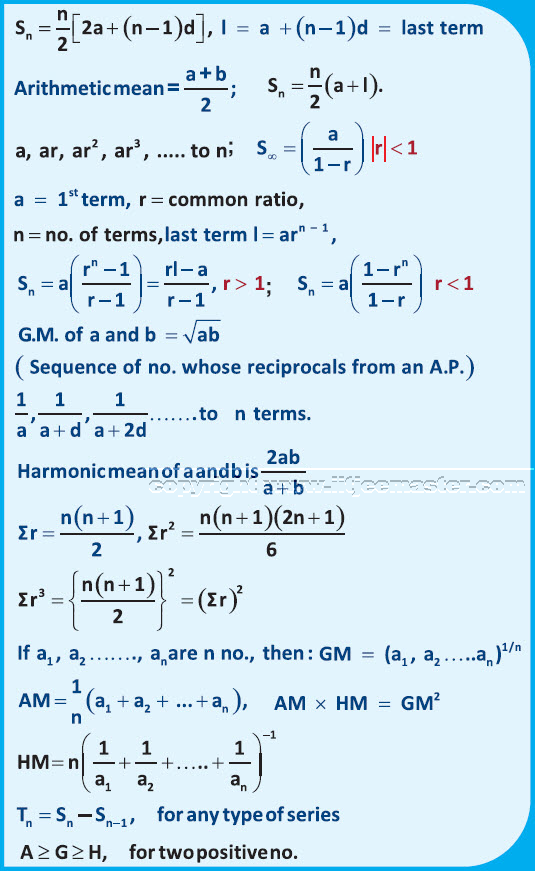In this video on JEE examination, we will be discussing about the topic sequence and series which is the third chapter of our syllabus. Now let us understand what is a sequence first. Basically, sequence implies the list of numbers in an order. Each number in the lists are called elements. Now suppose as per some rule, you write the following pattern of number like 1,3,5,7,9 etc or 2,4,8, 16 etc or you write 1,2,3,4,5 etc. These numbers have been written based on some rule such that each number is related to the other number.

Just like first number is related to second, second is related to third and so on. This relation can be anything such as an addition, division, multiplication, reciprocal etc. When these all are written, as per some rule, then they are known as a sequence. On the other hand, if we add them such as 1+3+6 etc or 2+4+6+8 etc then they are called as series.

Hence, they are all series together. We must see as to in how many forms we can classify or divide them or distribute them

Apart from the name sequence, it can also be called as a progression. On the other hand, series can also be known as sum of progression. Hence, we can see how many progressions are there. Arithmetic progression is an arithmetic progression which is a sequence whose terms increases or decreases by a fixed number. This fixed number is called common difference.

JEE maths examination videos are designed to assist you in giving detailed understanding of the topic. Apart from this all the concepts are backed by supporting illustrations to give you a clear understanding of the concept. Many a times students do not perform well due to lack of understanding of the concepts. However, you will find no such difficulty in understanding these topics. In these video lectures for jee maths, simple and interactive language is used to give you personalized training approach.

In these JEE examination video lectures, our efforts are to make you understand the concepts theoretically as well as practically. Professional exams are not that easy to crack but with our video lectures it will be much easier for you.

Also, try to maintain the flow of understanding in each of the video lectures. In case you are unable to understand any concept, you can always rewind the video lecture and watch it again.